# CGH: 2D Rectangular Apertures that are Parallel to the Image Screen

This chapter is intended primarily to replicate §I.B from the online class notes — see also an updated Table of Contents — that I developed in conjunction with a course that I taught in 1999 on the topic of Computer Generated Holography (CGH) for a subset of LSU physics majors who were interested in computational science. This discussion parallels the somewhat more detailed one presented in §I.A on the one-dimensional aperture oriented parallel to the image screen.

## Utility of FFT Techniques

Consider the amplitude (and phase) of light that is incident at a location (x1, y1) on an image screen that is located a distance Z from a rectangular aperture of width w and height h. By analogy with our accompanying discussion in the context of 1D apertures, the complex number, A, representing the light amplitude and phase at (x1, y1) will be,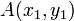$~A(x_1, y_1)$$~=$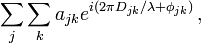$~ \sum_j \sum_k a_{jk} e^{i(2\pi D_{jk} /\lambda + \phi_{jk})} \, ,$

where, here, the summations are taken over all "j,k" elements of light across the entire 2D aperture, and now the distance Djk is given by the expression,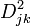$~D^2_{jk}$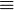$~\equiv$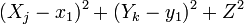$~ (X_j - x_1)^2 + (Y_k - y_1)^2 + Z^2$$~=$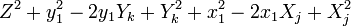$~ Z^2 + y_1^2 - 2y_1 Y_k + Y_k^2 + x_1^2 - 2x_1 X_j + X_j^2$$~=$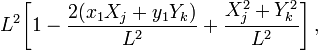$~ L^2 \biggl[1 - \frac{2(x_1 X_j + y_1 Y_k ) }{L^2} + \frac{X_j^2 + Y_k^2}{L^2} \biggr] \, ,$

and,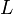$~L$$~\equiv$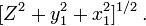$~ [Z^2 + y_1^2 + x_1^2]^{1 / 2} \, .$

If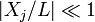$~|X_j/L| \ll 1$ and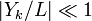$~|Y_k/L| \ll 1$ we can drop the quadratic terms in favor of the linear ones in the expression for Djk and deduce that,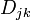$~D_{jk}$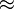$~\approx$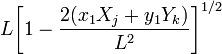$~ L \biggl[1 - \frac{2(x_1 X_j + y_1 Y_k ) }{L^2} \biggr]^{1 / 2}$$~\approx$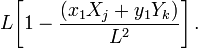$~ L \biggl[1 - \frac{(x_1 X_j + y_1 Y_k ) }{L^2} \biggr] \, .$

Hence, the double-summation expression for the amplitude at screen location (x1, y1) becomes,$~A(x_1, y_1)$$~\approx$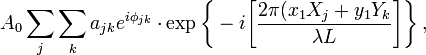$~ A_0 \sum_j \sum_k a_{jk} e^{i\phi_{jk} } \cdot \exp\biggl\{ -i \biggl[ \frac{2\pi(x_1 X_j + y_1Y_k}{\lambda L} \biggr] \biggr\} \, ,$

where,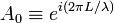$~A_0 \equiv e^{i(2\pi L/\lambda)}$.

When written in this form, it should be apparent why discrete Fourier transform techniques — specifically, 2D-FFT techniques — are useful tools for evaluation of the complex amplitude, A(x1, y1).

NOTE: If x1 and/or y1 are ever comparable in size to Z — which may be the case for large apertures or for apertures tilted by nearly 90° to the image screen — then the variation of L with image screen position cannot be ignored and, accordingly, the coefficient A0 cannot be moved outside of the double summation.

## Analytic Results

Now, as in §I.A, we would like to consider the case where the rectangular aperture is divided into an infinite number of divisions in both the X and Y dimensions and convert the summations in this last expression into integrals whose limits in both directions are given by the edges of the aperture. If we specifically consider the case where the aperture is assumed to be uniformly bright (i.e., ajk = a0 dX DY, and a0 is the brightness per unit area), and the phase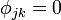$~\phi_{jk} = 0$ at all locations on the aperture, we can write,$~A(x_1, y_1)$$~\approx$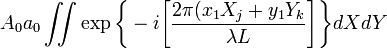$~ A_0 a_0 \iint \exp\biggl\{ -i \biggl[ \frac{2\pi(x_1 X_j + y_1Y_k}{\lambda L} \biggr] \biggr\} dX dY$$~=$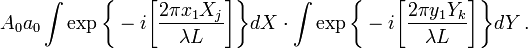$~ A_0 a_0 \int \exp\biggl\{ -i \biggl[ \frac{2\pi x_1 X_j }{\lambda L} \biggr] \biggr\} dX \cdot \int \exp\biggl\{ -i \biggl[ \frac{2\pi y_1Y_k}{\lambda L} \biggr] \biggr\} dY \, .$

Both of these integrals can be completed in the same fashion as described in §I.A for the 1D slit, giving,$~A(x_1, y_1)$$~\approx$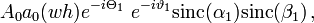$~ A_0 a_0 (wh) e^{-i\Theta_1} ~ e^{-i\vartheta_1} \mathrm{sinc}(\alpha_1) \mathrm{sinc}(\beta_1) \, ,$

where,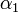$~\alpha_1$$~\equiv$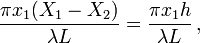$~\frac{\pi x_1 (X_1 - X_2)}{\lambda L}= \frac{\pi x_1 h}{\lambda L} \, ,$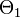$~\Theta_1$$~\equiv$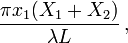$~\frac{\pi x_1 (X_1 + X_2)}{\lambda L} \, ,$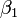$~\beta_1$$~\equiv$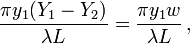$~\frac{\pi y_1 (Y_1 - Y_2)}{\lambda L}= \frac{\pi y_1 w}{\lambda L} \, ,$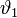$~\vartheta_1$$~\equiv$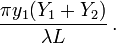$~\frac{\pi y_1 (Y_1 + Y_2)}{\lambda L} \, .$

It is worth noting that this derivation closely parallels the one presented in §8.5.1 (p. 393) of Born & Wolf (1980) (see the reference below). Specifically, our double-integral expression is identical to the "Fraunhofer diffraction integral" written down by Born & Wolf at the beginning of their §8.5.1; and if we follow Born & Wolf's lead and position the origin of our coordinate system at the center of the rectangle, then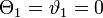$~\Theta_1 = \vartheta_1 = 0$ and the intensity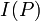$~I(P)$ at point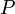$~P$ that is given by their equation (1) precisely matches the expression for the square of the amplitude,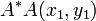$~A^*A(x_1, y_1)$, that is obtained from our last expression.